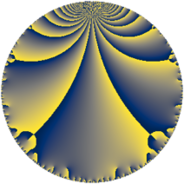# Properties

 Label 35.5.iLevel $35$ Weight $5$ Character orbit 35.i Rep. character $\chi_{35}(19,\cdot)$ Character field $\Q(\zeta_{6})$ Dimension $28$ Newform subspaces $1$ Sturm bound $20$ Trace bound $0$

# Related objects

## Defining parameters

 Level: $$N$$ $$=$$ $$35 = 5 \cdot 7$$ Weight: $$k$$ $$=$$ $$5$$ Character orbit: $$[\chi]$$ $$=$$ 35.i (of order $$6$$ and degree $$2$$) Character conductor: $$\operatorname{cond}(\chi)$$ $$=$$ $$35$$ Character field: $$\Q(\zeta_{6})$$ Newform subspaces: $$1$$ Sturm bound: $$20$$ Trace bound: $$0$$

## Dimensions

The following table gives the dimensions of various subspaces of $$M_{5}(35, [\chi])$$.

Total New Old
Modular forms 36 36 0
Cusp forms 28 28 0
Eisenstein series 8 8 0

## Trace form

 $$28 q + 94 q^{4} - 30 q^{5} - 222 q^{9} + O(q^{10})$$ $$28 q + 94 q^{4} - 30 q^{5} - 222 q^{9} + 390 q^{10} + 34 q^{11} - 258 q^{14} + 60 q^{15} - 206 q^{16} + 366 q^{19} - 1116 q^{21} - 288 q^{24} + 40 q^{25} + 474 q^{26} + 856 q^{29} - 1620 q^{30} - 5520 q^{31} - 2620 q^{35} + 11892 q^{36} + 1656 q^{39} + 6510 q^{40} - 1082 q^{44} + 7920 q^{45} - 5442 q^{46} + 8356 q^{49} - 15960 q^{50} - 2976 q^{51} + 4392 q^{54} + 11640 q^{56} - 13920 q^{59} - 5700 q^{60} - 24960 q^{61} - 45596 q^{64} + 5290 q^{65} + 10332 q^{66} - 26400 q^{70} + 38272 q^{71} + 31806 q^{74} + 41310 q^{75} + 22028 q^{79} + 48000 q^{80} - 29238 q^{81} + 62784 q^{84} - 33820 q^{85} - 21180 q^{86} - 12516 q^{89} + 4448 q^{91} - 89418 q^{94} - 28890 q^{95} - 27900 q^{96} - 3108 q^{99} + O(q^{100})$$

## Decomposition of $$S_{5}^{\mathrm{new}}(35, [\chi])$$ into newform subspaces

Label Dim. $$A$$ Field CM Traces $q$-expansion
$$a_2$$ $$a_3$$ $$a_5$$ $$a_7$$
35.5.i.a $$28$$ $$3.618$$ None $$0$$ $$0$$ $$-30$$ $$0$$